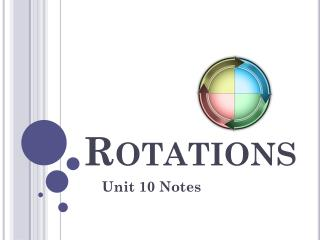# Rotations - PowerPoint PPT PresentationDownload PresentationRotations

RotationsDownload Presentation## Rotations

- - - - - - - - - - - - - - - - - - - - - - - - - - - E N D - - - - - - - - - - - - - - - - - - - - - - - - - - -
##### Presentation Transcript

1. Rotations Unit 10 Notes

2. A rotation is a transformation in which a figure is turned about a fixed point. The fixed point is called the center of rotation. Rays drawn from the center of rotation to a point and its image form an angle called the angle of rotation. The angle of rotation can be measured clockwise (CW) or counterclockwise (CCW).

3. Can you describe the rotation? Is there only one way to describe the rotation?

4. A figure in the plane has rotational symmetry if the figure can be mapped onto itself by a rotation of 180° or less.

5. A rotation is an isometry!

6. Rotations about the origin 90° CCW about the origin 270˚ CW

7. 180° CW or CCW about the origin

8. 270° CCW or 90˚CW about the origin

9. Practice #1 Rotate ∆ABC with vertices A(2,-1) , B(4,1), and C(3,3) by 90° about the origin.

10. Practice #2 Rotate ∆ABC with vertices A(2,-1) , B(4,1), and C(3,3) by 180° about the origin.

11. Practice #3 B 90˚ CW rotation of about X 180˚ CCW rotation of about X 45˚ CCW rotation of ΔAXB A C X D H G E F

12. Classwork and Homework Handout 7.3A and 7.3B